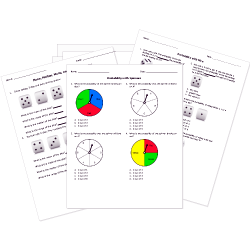Tweet# Free Printable Statistics and Probability WorksheetsThe ability to estimate, interpret, and manipulate data is an essential skill for statistics and probability. Strengthen student understanding of probability and statistics concepts with these printable probability worksheets and statistics resources.

Worksheets labeled with are accessible to Pro subscribers only. Become a Subscriber to access hundreds of premium worksheets.

Don't see a printable probability worksheet you need? Use our Test Maker™ to create your own statistics and probability printable that fits your needs. Browse statistics and probability questions or use our advanced search to find existing questions while filtering by grade levels and keywords. You can also create your own questions.

Related Lessons: Basic Probability and Mean, Median, Mode, and Range